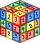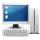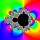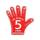GP - 8 items

Determine the first eight members of a geometric progression if a9=512, q=2

Result

a1 =  2
a2 =  4
a3 =  8
a4 =  16
a5 =  32
a6 =  64
a7 =  128
a8 =  256

Solution:Leave us a comment of example and its solution (i.e. if it is still somewhat unclear...):Be the first to comment!Next similar examples:

1. Five membersWrite first 5 members geometric sequence and determine whether it is increasing or decreasing: a1 = 3 q = -2
2. QuotientDetermine the quotient and the second member of the geometric progression where a3=10, a1+a2=-1,6 a1-a2=2,4.
3. CoefficientDetermine the coefficient of this sequence: 7.2; 2.4; 0.8
4. Geometric sequence 4It is given geometric sequence a3 = 7 and a12 = 3. Calculate s23 (= sum of the first 23 members of the sequence).
5. Geometric sequence 5About members of geometric sequence we know: ? ? Calculate a1 (first member) and q (common ratio or q-coefficient)
6. Six termsFind the first six terms of the sequence a1 = -3, an = 2 * an-1
7. A perineumA perineum string is 10% shorter than its original string. The first string is 24, what is the 9th string or term?
8. GP membersThe geometric sequence has 10 members. The last two members are 2 and -1. Which member is -1/16?
9. Tenth memberCalculate the tenth member of geometric sequence when given: a1=1/2 and q=2
10. Geometric progression 2There is geometric sequence with a1=5.7 and quotient q=-2.5. Calculate a17.
11. SequenceFind the common ratio of the sequence -3, -1.5, -0.75, -0.375, -0.1875. Ratio write as decimal number rounded to tenth.
12. Geometric progression 48,4√2,4,2√2
13. ComputerThe computer was purchased 10000,-. Each year, the price of a computer depreciates always the same percentage of the previous year. After four years, the value of the computer is reduced to 1300,- How many percent was depreciated price of the computer each
14. Geometric progressionFill 4 numbers between 4 and -12500 to form geometric progression.
15. Theorem proveWe want to prove the sentence: If the natural number n is divisible by six, then n is divisible by three. From what assumption we started?
16. ValueFind the value of the expression: 6!·10^-3
17. CalculationHow much is sum of square root of six and the square root of 225?